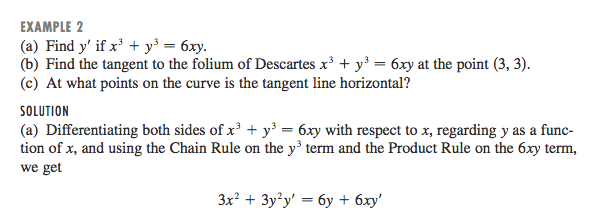# Differentiating Descartes' folium

## Homework Statement## The Attempt at a Solution

When the textbook says: Differentiating both sides of x^3 + y^3 = 6xy with respect to x, regarding y as a function of x, and using the Chain Rule on the y^3 term and the Product Rule on the 6xy term, we get

I really don't get what they're saying. I understand chain rule and product rule, but where the y' and the 6xy comes from I don't know.

## Answers and Replies

Dick
Science Advisor
Homework Helper
Take y to be a function of x. You don't understand why e.g. d/dx(y(x)^3)=3*y(x)^2*dy(x)/dx? Which is (y^3)'=3y^2*y'. That's just the chain rule.

Last edited:
When the textbook says: Differentiating both sides of x^3 + y^3 = 6xy with respect to x, regarding y as a function of x, and using the Chain Rule on the y^3 term and the Product Rule on the 6xy term, we get

I really don't get what they're saying. I understand chain rule and product rule, but where the y' and the 6xy comes from I don't know.

If z = f(x) and x = f(y), then dz/dy = [dz/dx]*[dx/dy]. This is the chain rule.

In this problem, let z = y^3. Then, d(y^3)/dx = [d(y^3)/dy]*[dy/dx] = (3y^2)*y'.

y^3 cannot be differentiated w.r.t. x, so the chain rule is used to rewrite the derivative so that y^3 can be differentiated w.r.t. y and an answer obtained.

As for the product rule, we know that if z(x) = u(x)*v(x), then dz/dx = [u(x)*(dv/dx)] + [v(x)*(du/dx)]. This is the product rule.

In this problem, let z(x) = 6xy, u(x) = 6x and v(x) = y(x). Then, d(6xy)/dx = [6x*(dy/dx)] + [y*6] = 6y + 6xy'.

Shoot any questions you might still have.

I consulted some youtube videos. I understand it now. Thanks for your help.